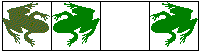The Toads And Frogs Puzzle is also known under the names of Hares and Tortoise and Sheep and Goats. With no animals at hand, it can be played with two kinds of coins. The following names still reflect on the essence of the activity: Hop, Skip, Jump and Traffic Jam.

N frogs are placed on N successive positions on the left of a string of squares; M toads occupy M rightmost squares. On the whole, there are M + N + 1 squares, so that just one square remains unoccupied.

Frogs only move rightward; toads move leftward. Every move is either a Slide to the nearby square or a Jump over one position, which is allowed only if the latter is occupied by a fellow of a different kind. In any case, no two animals are allowed in the same square.

The goal is to move toads into M leftmost positions and the frogs into N rightmost positions.

The number of toads and frogs can change between 1 and 5. Originally, N = M = 3. These are shown just above the string of squares. To change the values, click on either a little off its center line.

(Click on a piece you wish to move.)

### Please try switching to IE 11 (Windows) or Safari (Mac), for no other browser nowadays runs Java applets. If asked whether to allow the applet to load, click Yes - the applet is signed with a security certificate from a trusted company. But, regardless, there is an explanation below.What if applet does not run?

For every combination of N and M, the puzzle has two solutions: one can start either towards right or left. In both cases, the total number of moves is MN + M + N. There are MN Jumps and M + N Slides.

There is another interesting fact. For every sequence of moves, we can form a string of letters S and J. S denotes a Slide, while J stands for a Jump. Because of the symmetry, there is no wonder that, when M = N, the two strings corresponding to the two solutions are identical. What's interesting is that the same is true even when M differs from N. In addition, the string of moves is always palindromic.

We can also look at the movements of the hole - the empty square. This can move either 1 (r) or 2 (R) places right, or 1 (l) or 2 (L) places left. Unless M = N, the string thus obtained is no longer palindromic. They are palindromic whenever M = N. Strings corresponding to the two solutions are also different. However, they are not unrelated!

At every stage of solution, when it comes to selecting the next move, you may have to select either between two jumps, or two slides or a jump and a slide. One approach to solving the puzzle is to develop a certain strategy of behavior in each of the three cases.

Once you figured out how to solve the puzzle, you may want to try a two dimensional variant.

### References

1. W.W. Rouse Ball and H.S.M. Coxeter, Mathematical Recreations and Essays, Dover, 1987
2. E.R. Berlekamp, J.H. Conway, R.K. Guy, Winning Ways for Your Mathematical Plays, v1, A K Peters, 2001.
3. E.R. Berlekamp, J.H. Conway, R.K. Guy, Winning Ways for Your Mathematical Plays, v2, Academic Press, 1982.

### On Internet### The number of moves

Each of the N frogs must move M + 1 positions to the right. Each of the M toads must move N + 1 positions to the left. The total number of positions to move over is thus given by N(M + 1) + M(N + 1). However, on a jump, a piece moves over 2 positions. How many jumps are there?

It's easier to think of jumps as orientation swaps. Who ever does the move, a pair of neighboring pieces frog-toad converts to the pair toad-frog. To reach the goal, each of the toads must swap orientation with each of the frogs. Therefore, there is the total of MN swaps, or jumps. Therefore, the number of moves needed to solve the problem is

N(M + 1) + M(N + 1) - MN = MN + M + N### Strategy

It's worthwhile to make several observations. These may be short and not well grounded, but can be varified by experimenting with the applet. The bottom line is that the right moves are actually forced: at every given moment (excluding the starting configuration) there is only one right move.

1. Note that

Jump/Jump is a fatal configuration:Whichever jump you choose, you are stuck on the next move. (Unless, of course, the "hole" moves to an end square. In which case you get stuck on the second move.)

2. If the choice is Jump/Slidealways choose a jump; for you'll stuck after a slide.

3. When it's a question of selecting between two slides, see that the configuration you are getting is not the fatal Jump/Jump.### Why is the sequence of moves palindromic?

To solve the puzzle is to move all frogs to the right and all toads to the left. When this is done, we can think of the result as having a new puzzle where backward-looking frogs became toads, and the backward-looking toads turned into forward-looking frogs. A sequence of moves that solved the original puzzle will solve the new puzzle if read backwards.

For every M and N, there are two solutions. One starts with a leftward slide, the other with a rightward slide. If M = N, then the two solutions form a "double helix": right moves in one correspond to left moves in the other, and vice versa. Combined with the observation of the previous paragraph, this explains why, when M = N, the sequence of moves is palindromic.

It's another observation that the sequence of moves seems to be palindromic even when M ≠ N. For this I do not have an explanation yet.